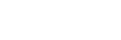# Digital Scientific Calculator

by Nathina

This is a calculator that is specifically designed to help with science, engineering, and math problems. The scientific calculator has way more buttons than your standard calculator. The standard calculator just allows you to perform your four basic arithmetic operations of addition, subtraction, multiplication, and division. Following are the steps to be followed for building the Digital Scientific Calculator, 1. Linear Algebra And Floating Point 2. Complex Number And Matrix 3. Big Number And Logarithm 4. Common Libraries For Build Calculator
• © 2022 Open Weaver Inc.